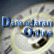##Dividend Regressions: January 2015

Variables used in the regression

1. Dividend Yield = Dividends per share in most recent year/ Current Stock Price
2. Dividend Payout Ratio = Dividends / Net Income
3. Beta: Regression or Bottom up beta
4. % of shares held by institutions = Percent of outstanding shares held by institutional investors
5. Expected Growth in EPS over next 5 years = Consensus analyst estimate (or your own) of expected growth in EPS . If you don't have an analyst estimate, use your own estimate of expected growth.
6. Market Debt to Capital = Debt/ (Debt + Market Value of Equity): If you have market value for debt, use it. If not, use book value of debt and market value of equity.

US Regression: Dividend Yield

 Model Summarya Model R R Square Adjusted R Square Std. Error of the Estimate 1 .483b .233 .231 1.43761 a. Broad Group = United States b. Predictors: (Constant), Beta, Market Debt to Capital Ratio, % held by institutions, Expected growth rate in EPS- Next 5 years

 Coefficientsa,b,c Model Unstandardized Coefficients Standardized Coefficients t Sig. B Std. Error Beta 1 (Constant) .048 .002 27.730 .000 % held by institutions -.021 .002 -.249 -10.099 .000 Expected growth rate in EPS- Next 5 years -.050 .005 -.229 -9.136 .000 Market Debt to Capital Ratio .012 .002 .161 6.558 .000 Beta -.008 .001 -.200 -7.634 .000 a. Broad Group = United States b. Dependent Variable: Dividend Yield c. Weighted Least Squares Regression - Weighted by Market Cap (in US \$)

US Regression: Dividend Payout

 Model Summarya Model R R Square Adjusted R Square Std. Error of the Estimate 1 .500b .250 .248 23.5862000 a. Broad Group = United States b. Predictors: (Constant), Beta, Market Debt to Capital Ratio, % held by institutions, Expected growth rate in EPS- Next 5 years

 Coefficientsa,b,c Model Unstandardized Coefficients Standardized Coefficients t Sig. B Std. Error Beta 1 (Constant) .835 .031 27.345 .000 % held by institutions -.205 .039 -.148 -5.315 .000 Expected growth rate in EPS- Next 5 years -.678 .104 -.185 -6.541 .000 Market Debt to Capital Ratio -.039 .032 -.034 -1.235 .217 Beta -.222 .019 -.341 -11.434 .000 a. Broad Group = United States b. Dependent Variable: Payout ratio c. Weighted Least Squares Regression - Weighted by Market Cap (in US \$)

Global Regression: Dividend Yield

 Model Summary Model R R Square Adjusted R Square Std. Error of the Estimate 1 .428a .183 .183 1.69314 a. Predictors: (Constant), ERP for Country, Market Debt to Capital Ratio, Expected growth rate in EPS- Next 5 years, % held by institutions

 Coefficientsa,b Model Unstandardized Coefficients Standardized Coefficients t Sig. B Std. Error Beta 1 (Constant) .040 .002 21.121 .000 % held by institutions -.018 .001 -.251 -18.913 .000 Expected growth rate in EPS- Next 5 years -.058 .002 -.287 -23.396 .000 Market Debt to Capital Ratio .018 .001 .187 15.230 .000 ERP for Country .000 .000 -.016 -1.192 .233 a. Dependent Variable: DivYield b. Weighted Least Squares Regression - Weighted by Market Cap (in US \$)

Global Regression: Dividend Payout

 Model Summary Model R R Square Adjusted R Square Std. Error of the Estimate 1 .335a .112 .112 20.1614961 a. Predictors: (Constant), Beta, Expected growth rate in EPS- Next 5 years, Market Debt to Capital Ratio, % held by institutions

 Coefficientsa,b Model Unstandardized Coefficients Standardized Coefficients t Sig. B Std. Error Beta 1 (Constant) .641 .011 57.561 .000 % held by institutions -.143 .011 -.185 -12.661 .000 Expected growth rate in EPS- Next 5 years -.533 .035 -.218 -15.345 .000 Market Debt to Capital Ratio -.054 .015 -.052 -3.566 .000 Beta -.067 .005 -.205 -13.685 .000 a. Dependent Variable: Payout ratio b. Weighted Least Squares Regression - Weighted by Market Cap (in US \$)
• How do I use this regression?

Assume that you want to estimate the dividend payout ratio for a firm with the following characteristics, using the US regression:

Institutional holdings= 75% of outstanding stock

Regression beta = 1.20

Expected Growth in EPS over next 5 years = 12%

Market Debt to Capital = 20%

• Predicted Value

Expected Dividend payout ratio= 0.835 - 0.205 (.75) - 678 (.12) - .039 (.20) -.222 (1.20) = .3257 or 32.57%

If your predicted value is less than zero, your predicted dividend payout ratio is zero.